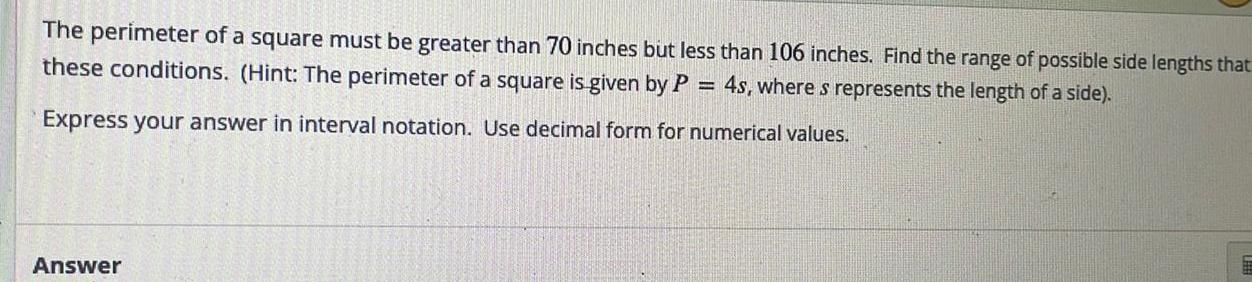Question:

# The perimeter of a square must be greater than 70 inches but

Last updated: 9/16/2023The perimeter of a square must be greater than 70 inches but less than 106 inches Find the range of possible side lengths that these conditions Hint The perimeter of a square is given by P 4s where s represents the length of a side Express your answer in interval notation Use decimal form for numerical values Answer